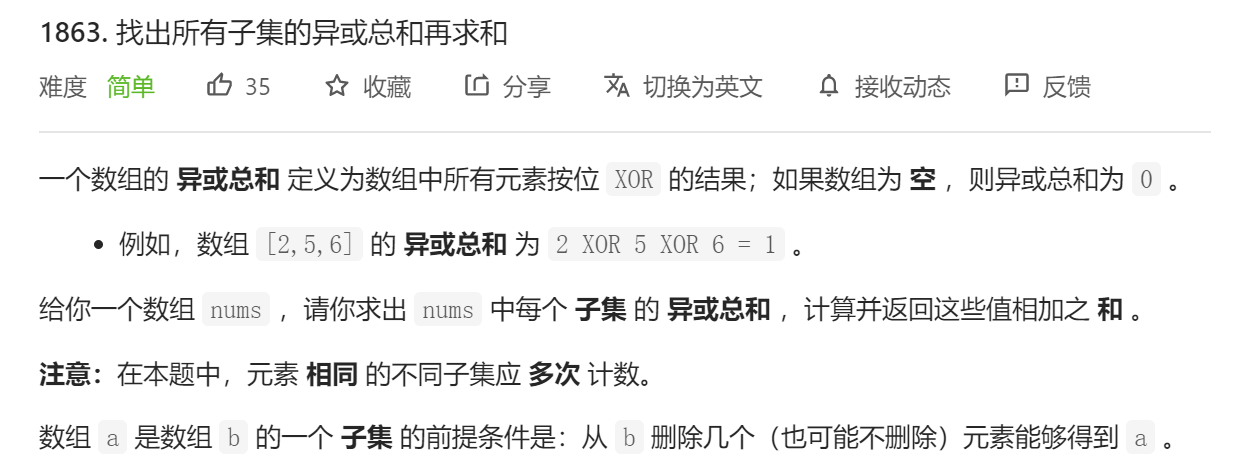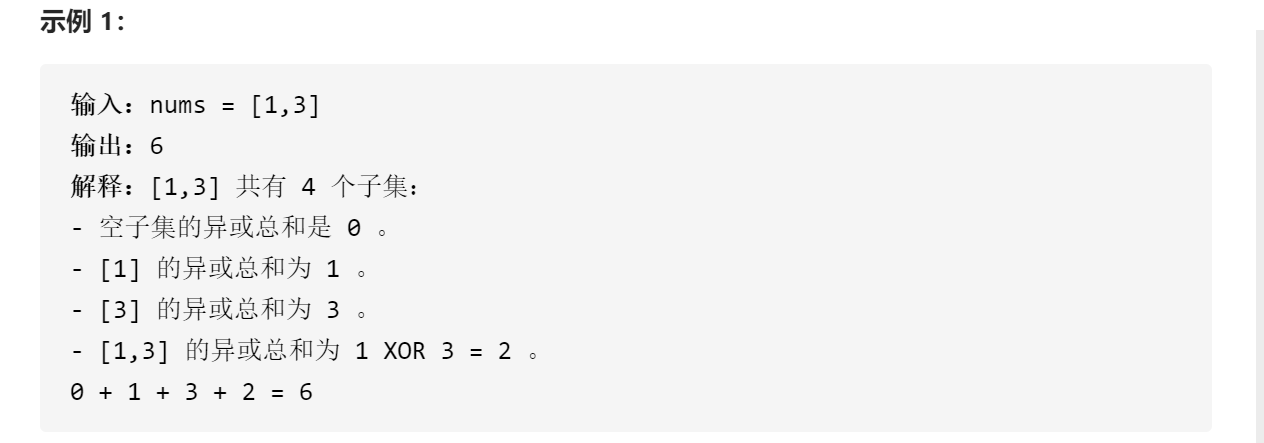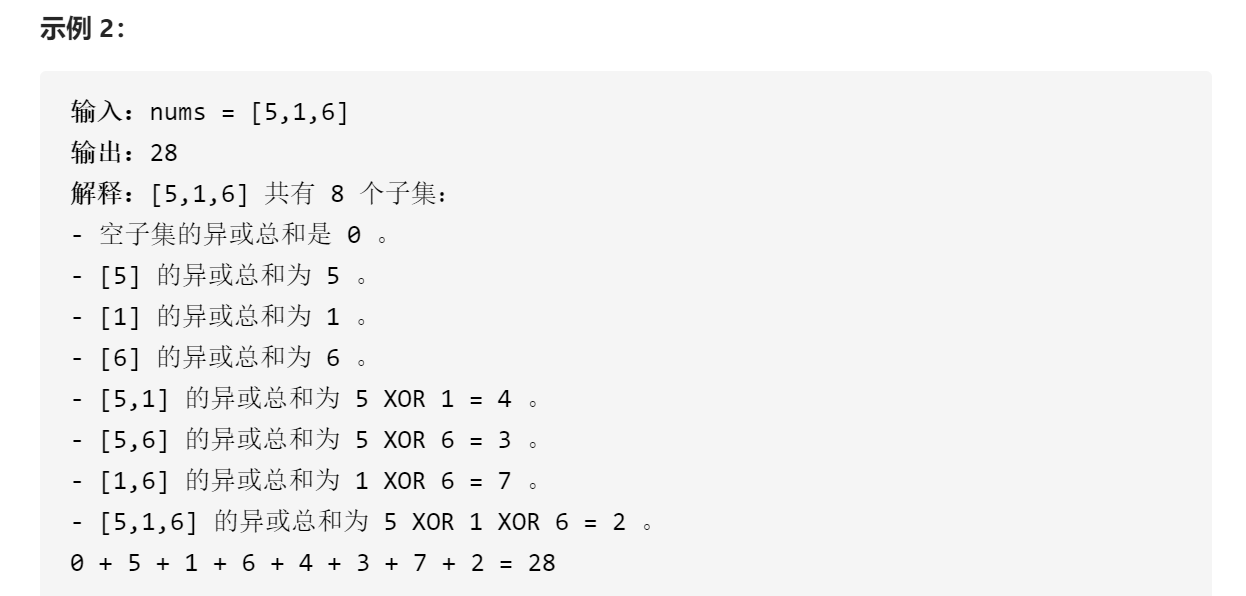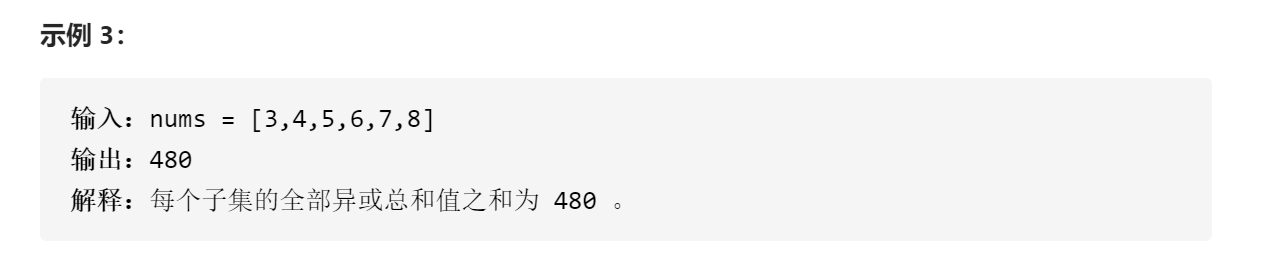### 一、题目描述### 二、示例### 三、条件限制### 四、解题思路及代码

#### 4.3 按位考虑 + 二项式展开

$Count=(C_K^1+C_K^3+C_K^5+\dots+C_K^m)*2^{N-K}$

$Count = 2^{K-1}*2^{N-K} = 2^{N-1}$